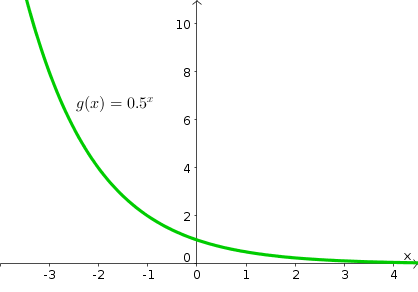# Help making a math function

Hello,

I am in need of help trying to make a exponential funtion.But I am lost on how to do it.

robloxapp-20220816-1408074.wmv (536.6 KB)
How would I get it to work like the one in this video?

There are three values im working with, “Cost”, “0-1”(On the scroll bar), and “Max Bag Space”

2 LikesA funtion like this would probably be better

Are you trying to get the Y by placing X?
If so, the following can get you the result:

``````function g(x : number)
return math.pow(0.5,x)
end
``````

Every X you write will give you the Y

If you’re referring to the decreasing intervals between each tick, I suppose you can put the result of g(x) in task.wait().
For example:

``````function g(x : number)
return math.pow(0.5,x)
end

local startX = 1
local endX = 8
local interval = 0.1

for x = startX, endX, interval do